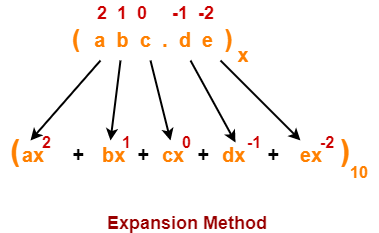## Number System Conversions-

Before you go through this article, make sure that you have gone through the previous article on Basics of Number System.

In number system,

• It is very important to have a good knowledge of how to convert numbers from one base to another base.
• Here, we will learn how to convert any given number from any base to base 10.## Converting to Base 10-

A given number can be converted from any base to base 10 using Expansion Method.

According to expansion method, if abc.de is any given number in base x, then its value in base 10 is given as-

(abc.de)x = (ax2 + bx + c + dx-1 + ex-2)10

### Explanation-

To use expansion method for conversion,

• Assign position number to each digit of the given number.
• Digits to the left of decimal are numbered starting from 0.
• Digits to the right of decimal are numbered starting from -1.
• Write a term for each digit as digit x (base of given number)position number of digit
• Perform the addition of all terms to obtain the number in base 10.
• This formula can be expanded for any number of digits.## PRACTICE PROBLEMS BASED ON CONVERSION TO BASE 10-

Convert the following numbers to base 10-

1. (10010)2
2. (254)8
3. (AC)16
4. (10010.101)2
5. (254.7014)8
6. (AC.FBA5)16
7. (0.1402)8
8. (0.ABDF)16

## Solutions-

### 1. (10010)2

#### (10010)2 → ( ? )10

Using expansion method, we have-

(10010)2

= ( 1 x 24 + 0 x 2+ 0 x 22 + 1 x 21 + 0 x 20 )10

= ( 16 + 0 + 0 + 2 + 0 )10

= ( 18 )10

### 2. (254)8

#### (254)8 → ( ? )10

Using expansion method, we have-

(254)8

= ( 2 x 82 + 5 x 8+ 4 x 80 )10

= ( 128 + 40 + 4 )10

= ( 172 )10

### 3. (AC)16

#### (AC)16 → ( ? )10

Using expansion method, we have-

(AC)16

= ( A x 161 + C x 160 )10

= ( 10 x 16 + 12 x 1 )10

= ( 160 + 12 )10

= ( 172 )10

### 4. (10010.101)2

#### (10010.101)2 → ( ? )10

Using expansion method, we have-

(10010.101)2

= ( 1 x 24 + 0 x 2+ 0 x 22 + 1 x 21 + 0 x 20 + 1 x 2-1 + 0 x 2-2 + 1 x 2-3 )10

= ( 16 + 0 + 0 + 2 + 0 + 0.5 + 0.125 )10

= ( 18.625 )10

### 5. (254.7014)8

#### (254.7014)8 → ( ? )10

Using expansion method, we have-

(254.7014)8

= ( 2 x 82 + 5 x 8+ 4 x 80 + 7 x 8-1 + 0 x 8-2 + 1 x 8-3 + 4 x 8-4 )10

= ( 128 + 40 + 4 + 0.875 + 0.0019 + 0.0009 )10

= ( 172.8778 )10

### 6. (AC.FBA5)16

#### (AC.FBA5)16 → ( ? )10

Using expansion method, we have-

(AC.FBA5)16

= ( A x 161 + C x 160 + F x 16-1 + B x 16-2 + A x 16-3 + 5 x 16-4 )10

= ( 10 x 16 + 12 x 1 + 15 x 16-1 + 11 x 16-2 + 10 x 16-3 + 5 x 16-4 )10

= ( 160 + 12 + 0.9375 + 0.0429 + 0.0024 + 0.0001 )10

= ( 172.9829 )10

### 7. (0.1402)8

#### (0.1402)8 → ( ? )10

Using expansion method, we have-

(0.1402)8

= ( 0 x 80 + 1 x 8-1 + 4 x 8-2 + 0 x 8-3 + 2 x 8-4 )10

= ( 0 + 0.125 + 0.0625 + 0 + 0.0005 )10

= ( 0.188 )10

### 8. (0.ABDF)16

#### (0.ABDF)16 → ( ? )10

Using expansion method, we have-

(0.ABDF)16

= ( 0 x 160 + A x 16-1 + B x 16-2 + D x 16-3 + F x 16-4 )10

= ( 0 x 1 + 10 x 16-1 + 11 x 16-2 + 13 x 16-3 + 15 x 16-4 )10

= ( 0 + 0.625 + 0.0429 + 0.0032 + 0.0002 )10

= ( 0.6713 )10

To gain better understanding about Conversion to Base 10,

Watch this Video Lecture

Next Article- Decimal to Binary Conversion

Get more notes and other study material of Number System.

Watch video lectures by visiting our YouTube channel LearnVidFun.